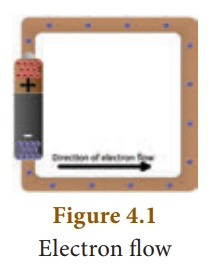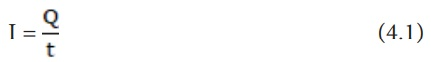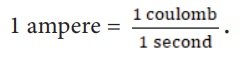Home | | Science 10th Std | Electric Current

# Electric Current

The motion of electric charges (electrons) through a conductor (e.g., copper wire) will constitute an electric current. This is similar to the flow of water through a channel or flow of air from a region of high pressure to a region of low pressure.

ELECTRIC CURRENT

The motion of electric charges (electrons) through a conductor (e.g., copper wire) will constitute an electric current. This is similar to the flow of water through a channel or flow of air from a region of high pressure to a region of low pressure.

In a similar manner, the electric current passes from the positive terminal (higher electric potential) of a battery to the negative terminal (lower electric potential) through a wire as shown in the Figure 4.1.## 1.DefinitionŌĆéofŌĆéelectriccurrent

Electric current is often termed as ŌĆścurrentŌĆÖ and it is represented by the symbol ŌĆśIŌĆÖ. It is defined as the rate of flow of charges in a conductor . This means that the electric current represents the amount of charges flowing in any cross section of a conductor (say a metal wire) in unit time. If a net charge ŌĆśQŌĆÖ passes through any cross section of a conductor in time ŌĆśtŌĆÖ, then the current flowing through the conductor is

time ŌĆśtŌĆÖ, then the current fl owing through the conductor is

I = Q/t## 2.SIŌĆéunitŌĆéofŌĆéelectricŌĆécurrent

The SI unit of electric current is ampere (A). The current flowing through a conductor is said to be one ampere, when a charge of one coulomb flows across any cross-section of a conductor, in one second. Hence,### Solved Problem-1

A charge of 12 coulomb flows through a bulb in 5 second. What is the current through the bulb?

### Solution:

Charge Q = 12 C, Time t = 5 s. Th erefore, current I = Q/t = 12/5 = 2.4 ATags : Definition and SI unit , 10th Science : Chapter 4 : Electricity
Study Material, Lecturing Notes, Assignment, Reference, Wiki description explanation, brief detail
10th Science : Chapter 4 : Electricity : Electric Current | Definition and SI unit